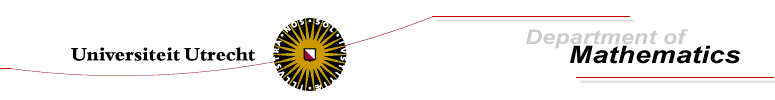Calculations

For my dissertation I have calculated many geometric structures using Maple. Below you will find a list of examples. If there are any questions about the packages or the calculations, do not hesitate to contact the author eendebak@math.uu.nl.

The Maple packages needed for these calculations can be downloaded here. The main packages are:

• jetpte. This is an extension to thet jets package. It contains routines to define a frame on a manifold and perform some basic calculations with this frame.
• MongeSystems is a Maple package to calculate the Monge systems of first order systems or second order scalar equations.
• Tableau is a Maple package to calculate prolongations of a tableau in the context of exterior differential systems.
• GeneralizedLaplace. A Maple package to calculate generalized Laplace sequences of second order partial differential equations.

A list of calculations for the thesis:

• first-order-system-invariant-coframe.mws. This worksheet contains the calculation of an invariant frame on the equation manifold of a first order system. Even though the equation is non-linear and the Nijenhuis tensor is generic up to third order the system has a 5 dimensional symmetry group.
• system-rankD-1.mws. This worksheet contains examples of hyperbolic first order systems for which the image D of the Nijenhuis tensor has rank 1. These examples are discussed in the chapter `Systems and equations with non-generic Nijenhuis tensor' of my dissertation.
• tangential-symmetries.mws. This worksheet contains the calculation of tangential symmetries for several Darboux integrable systems. The systems are examples in the chapter `Tangential symmetries' (Chapter 10) of my dissertation.
• Example 8.2.8. Calculation of the Monge systems and an adapted coframing for the hyperbolic first order system q=r, p+q^2/2=0.